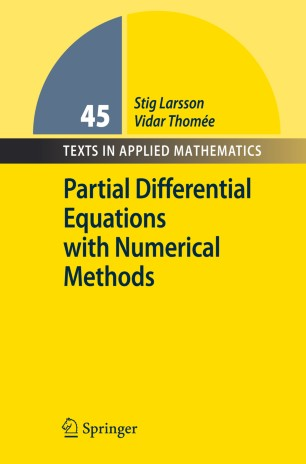# Partial Differential Equations with Numerical Methods

• Very well-written

• Elementary introductionTextbook

Part of the Texts in Applied Mathematics book series (TAM, volume 45)

1. Front Matter
Pages I-XI
2. Pages 1-14
3. Pages 15-24
4. Pages 25-41
5. Pages 77-94
6. Pages 109-127
7. Pages 129-148
8. Pages 149-161
9. Pages 163-183
10. Pages 185-199
11. Pages 201-216
12. Pages 217-224
13. Back Matter
Pages 225-261

### Introduction

The book is suitable for advanced undergraduate and beginning graduate students of applied mathematics and engineering. The main theme is the integration of the theory of linear PDEs and the numerical solution of such equations. For each type of PDE, elliptic, parabolic, and hyperbolic, the text contains one chapter on the mathematical theory of the differential equation, followed by one chapter on finite difference methods and one on finite element methods. As preparation, the two-point boundary value problem and the initial-value problem for ODEs are discussed in separate chapters. There is also one chapter on the elliptic eigenvalue problem and eigenfunction expansion. The presentation does not presume a deep knowledge of mathematical and functional analysis. Some background on linear functional analysis and Sobolev spaces, and also on numerical linear algebra, is reviewed in two appendices.

### Keywords

Boundary value problem Sobolev space elliptic equation finite difference method finite element method hyperbolic equation parabolic equation partial differential equation

#### Authors and affiliations

1. 1.Department of Mathematics SciencesChalmers University of Technology and University of GothenburgGöteborgSweden

### Bibliographic information

• Book Title Partial Differential Equations with Numerical Methods
Vidar Thomee
• Series Title Texts in Applied Mathematics
• DOI https://doi.org/10.1007/978-3-540-88706-5
• Copyright Information Springer Berlin Heidelberg 2003
• Publisher Name Springer, Berlin, Heidelberg
• eBook Packages
• Hardcover ISBN 978-3-540-01772-1
• Softcover ISBN 978-3-540-88705-8
• eBook ISBN 978-3-540-88706-5
• Series ISSN 0939-2475
• Edition Number 1
• Number of Pages X, 262
• Number of Illustrations 0 b/w illustrations, 0 illustrations in colour
• Topics
• Buy this book on publisher's site
Industry Sectors
Aerospace
IT & Software

## Reviews

From the reviews:

"The book under review is an introduction to the field of linear partial differential equations and to standard methods for their numerical solution. … The balanced combination of mathematical theory with numerical analysis is an essential feature of the book. … The book is easily accessible and concentrates on the main ideas while avoiding unnecessary technicalities. It is therefore well suited as a textbook for a beginning graduate course in applied mathematics." (A. Ostermann, IMN - Internationale Mathematische Nachrichten, Vol. 59 (198), 2005)

"This book, which is aimed at beginning graduate students of applied mathematics and engineering, provides an up to date synthesis of mathematical analysis, and the corresponding numerical analysis, for elliptic, parabolic and hyperbolic partial differential equations. … This widely applicable material is attractively presented in this impeccably well-organised text. … Partial differential equations with numerical methods covers a lot of ground authoritatively and without ostentation and with a constant focus on the needs of practitioners." (Nick Lord, The Mathematical Gazette, March, 2005)

"Larsson and Thomée … discuss numerical solution methods of linear partial differential equations. They explain finite difference and finite element methods and apply these concepts to elliptic, parabolic, and hyperbolic partial differential equations. … The text is enhanced by 13 figures and 150 problems. Also included are appendixes on mathematical analysis preliminaries and a connection to numerical linear algebra. Summing Up: Recommended. Upper-division undergraduates through faculty." (D. P. Turner, CHOICE, March, 2004)

"This book presents a very well written and systematic introduction to the finite difference and finite element methods for the numerical solution of the basic types of linear partial differential equations (PDE). … the book is very well written, the exposition is clear, readable and very systematic." (Emil Minchev, Zentralblatt MATH, Vol. 1025, 2003)

"The author’s purpose is to give an elementary, relatively short, and readable account of the basic types of linear partial differential equations, their properties, and the most commonly used methods for their numerical solution. … We warmly recommend it to advanced undergraduate and beginning graduate students of applied mathematics and/or engineering at every university of the world." (Ferenc Móricz, Acta Scientiarum Mathematicarum, Vol. 71, 2005)

"The presentation of the book is smart and very classical; it is more a reference book for applied mathematicians … . The convergence results, error estimates, variation formulations, all the theorems proofs, are very clear and well presented, the annexes A and B summary the necessary background for the understanding, without redundant generalisation or forgotten matter. The bibliography is presented by theme, well targeted on the topic of the book." (Anne Lemaitre, Physicalia Magazine, Vol. 28 (1), 2006)

“Offers basic theory of linear partial differential equations and discusses the most commonly used numerical methods to solve these equations. … There are two appendices providing some extra basic material, useful to help understanding some of the theoretical principles that might be unfamiliar to unexperienced readers and students. The text is elementary and meant for students in mathematics, physics, engineering. … The bibliography is well arranged according to the important issues, which makes it easy to get informed about possible references for further study.” (Paula Bruggen, Bulletin of the Belgian Mathematical Society, Vol. 15 (1), 2008)